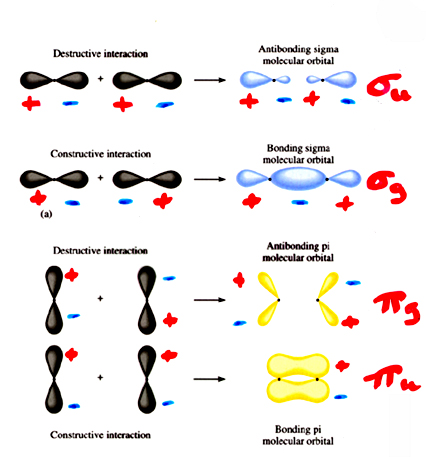# What are some examples of bonding orbitals?

The ${\sigma}_{u , 2 s}$ (or ${\sigma}_{2 s}^{\text{*}}$) is called a 2s sigma ungerade antibonding orbital. If you invert it (flip x and y coordinates in the xy plane), the lobes have opposite charges. Ungerade means odd.
Another example is a ${\pi}_{u , 2 {p}_{x , y}}$ (or ${\pi}_{2 {p}_{x , y}}^{\text{*}}$) orbital, which is a bonding orbital.Look at the "Antibonding sigma molecular orbital" for the ${\sigma}_{u , 2 s}$ and the "Bonding pi molecular orbital" for the ${\pi}_{u , 2 {p}_{x , y}}$.